## //----------------------------// ArtMatic 4 in 4 out components //----------------------------//

Introduction
Alpha scale & offset
XYZW Offset
XYZ Scale
3D Rotate
Smooth floor
S-Space Scale
S-Space Transforms #
S-Sphere Invert+offset
S-Perspective distort
S-Polar space
RGB * alpha
Color Shift
Contrast & offset
Color Controls #
(xziy) City Textures #
VY Themes Filters #
Memory w Logic #
Memory w Maths #
Memory w Alpha
Packed index (w) Mixer
44 Compiled tree

## Introduction

44 components are generally used to process RGB+Alpha or 3D space + Alpha information (Remember Alpha can hold (channel/elevation/DF field). They can be quite similar to 33 or 34 components. The output is generally of the same type as input. In this chapter, the left three inputs and outputs may be called either x y z or RGB. The fourth input and output may be called either alpha or w. In general we use X and Y capital case to refer to vector values and x y lower case to refer to scalar coordinates.
Some 44 components can be useful for modifying the RGB with the alpha channel value to implement various shaders like Alpha Fade or Alpha gradient. In many cases, the fourth input is passed through to the fourth output without change.
A bunch of 44 components serves as transforming a 3D space while maintaining a space scaling information in w as in the S-Space cases.

An essential in-depth discussion of ArtMatic structures Trees and components is found in ArtMatic Designer References and in Building trees.

##44 Alpha scale & offset

parameters :
A : Scale xyz (0. : 4.)
B : Scale w (alpha) (-4. : 4.)
C : Offset w (alpha) (-4. : 4.)

##44 XYZW Offset

parameters :
A : Offset x (-32. : 32.)
B : Offset y (-32. : 32.)
C : Offset z (-32. : 32.)
D : Offset w (-32. : 32.)

discussion :
Add independent offsets to the (x,y,z,w) inputs. It functions identically to 33 3D Offset. The fourth input should generally be kept unmodified.

##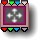44 XYZ Scale

parameters :
A : Scale X (-4. : 4.)
B : Scale Y (-4. : 4.)
C : Scale Z (-4. : 4.)

discussion :
Scale the (x,y,z) inputs using the vector (A,B,C) . The fourth input is passed out unchanged. This is functionally identical to the 3D Scale component. Infinity passes through unchanged.

##44 3D Rotate

parameters :
A : Angle xy (oZ) (-180. : 180.)
B : Angle xz (oY) (-180. : 180.)
C : Angle yz (oX) (-180. : 180.)

discussion :
This is simply a 3D Rotate (like 33 3D Rotate) with a fourth input that is passed through without influencing anything. This behavior is convenient when working with the various S-Space components.

algorithms :
• Rotate 3 axes :
• Rotate yz(oX) & Offset z & x
• Rotate xz(oY) & Offset x & y
• Rotate xy(oZ) & Offset y & z

##44 Smooth floor

parameters :
A : Floor level (-16. : 4.)
B : Roof level (0. : 100.)
C : Smoothness % (0. : 4.)

discussion :
Apply a Smooth clamping function to the alpha channel (fourth input) and pass through the other channels unchanged.'Roof level' has to be higher than 'Floor level'. See also the
11 Smooth Clamps component. The output is clipped to a valid color range (i.e. no negative values are passed out). Infinities in RGB are shaded with the Depth cue color in any cases.
Options 'Pass infinity' and 'Discard infinity'. When 'Discard infinity' is set infinity in alpha are mapped to -1. Discarding infinities might be necessary to avoid discontinuities in iterative fractal shaders or to discard the implicit infinity masking.

##44 S-Space Scale

parameters :
A : Scale (-50. : 50.)

discussion :
Scale the space uniformly and send out an adjusted scale value in output 4. This component takes a 3D space (xyz) plus a scale value as its inputs (such as the values generated by the
34 S-Space Scale component. The output is the scaled space (from the xyz outputs) and the fourth input (S) multiplied by the 'Scale' parameter value. In other words it outputs the vector (Scale*x,Scale*y,Scale*z,Scale*S).
The scale 'S' value is important for DFRM trees that distorts space to make sure that the system converges correctly ( Divide the Distance field value by the S-Space scale 4th output).

##44 S-Space Transforms #

parameters :
A : Scale (-4. : 4.)
B : Offset x (-32. : 32.)
C : Offset y (-32. : 32.)
D : Offset z (-32. : 32.)

discussion :
This component implements many interesting non-linear space transforms that would normally be challenging for DFRM (Distance Field Ray-Marching). Many of these are mind-bending transforms especially useful for creating 3D fractals. Most of the algorithms result in non-uniform space scaling and require use of the S (fourth) output to adjust the scaling of DF fields to avoid convergence problems.Example: A fractal obtained with "Rotation Sym xz & Spherical fold"algorithms :
• Scale & Offset :
• Abs & Scale & Offset :
• Space inversion :
Similar to the 22 Complex Inverse component. Inputs (x,y,z) AND S are divided by length(x,y,z)2. Parameter options : Radius 1, Radius Pi, Radius Pi2, Radius 16.Similar to the 22 Complex Inverse component. Inputs (x,y,z) AND S are divided by length(x,y,z) squared.
• Spherical fold :
Reflect the space between two spheres of Radius A and B where A and B are the values of the parameter sliders A and B.
• Sphere reflection :
• Mandelbox transform :
• Mirror N-Fold xy & Spherical fold :
Options: N-Fold 3 through 20. A mix of planar rotation symmetries with spherical folding.
• Mirror N-Fold xz & Spherical fold :
Options: N-Fold 3 through 20. A mix of planar rotation symmetries with spherical folding.
• 3D N-Fold oY oX :
Two planar rotation symmetries around they given axis.
• Tetra s-inversions :
• Cross s-inversions :
• Kleinian Box s-inversions :
Spherical inversion sets.
• BoxFold & s-inversions :

• Sierpinsky Tetrahedron :
45° mirror transform that converges to Sierpinsky Tetrahedron fractals when iterated.
• Sierpinsky Octahedron (x) :
45° mirror transform that converges to Sierpinsky Octahedron fractals when iterated.
• Dodecahedron Mirror(z+) :
• Octahedron Mirror (xyz+) :
• Box Mirror Tower (z+) :
• Saw Plane (xz) :
Infinite mirror saw and scaling in 2D on the xz plane.
• Saw Space
Infinite mirror saw and scaling in 3D. When iterated with rotation and offset, Saw Space creates interesting fractal textures and can be used to modify other objects.
• Boxodron (x+) :
• Pyranodron (yz+) :
• Pyrabulbodron (yz+) :

##44 S-Sphere Invert+offset

parameters :
A : Radius (1. : 16.)
B : Offset x (-32. : 32.)
C : Offset y (-32. : 32.)
D : Offset z (-32. : 32.)

discussion :
This component reflects the space around a sphere. The scaling is non-linear; so, make sure to use the s-output (the fourth) to divide the DF amplitude at the end of the tree to avoid convergence problems. S-Sphere Invert+offset is typically used in conjunction with the
S-Space Scale component.

Example: The component's s-output needs to be used to scale the DF object field. In this example, the compiled tree creates a color DF object (RGBA). The input is a 3D space in the xyz inputs and a scale value in the fourth input. The output is a 3D space plus a scale value :##44 S-Perspective distort

parameters :
A : Amount (0. : 1.)

##44 S-Polar space

parameters :
A : Radial Scale (1. : 100.)
B : Dist Scale (0.12 : 1.)
C : Dist Offset (-16. : 64.)
D : Radial Offset (-32. : 32.)

• "orient oY ,use x",
• "orient oY ,use |x|", //absolute value of X
• "orient oY ,use -|x|",
• "orient oZ ,use x",
• "orient oZ ,use |x|",
• "orient oZ ,use -|x|",

##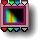44 RGB * alpha

parameters :
A : Alpha contrast (0. : 25.)
B : Highlights level (0. : 2.)
C : Alpha level (-4. : 4.)

discussion :
Multiply the R, G and B channels with the w/alpha channel. The w/alpha input is passed out unchanged through the fourth input. This is often very useful for helping visualize the alpha channel. 'Alpha Contrast' controls the contrast of the alpha channel before it is multiplied with RGB. 'Highlights Level' provides highlights where the alpha channel amplitude is large. This parameter controls the brightness of the highlights. These are added on top of the RGB.
"Alpha Level" is a scaling factor applied to the alpha before the alpha is multiplied with the RGB.

Options:

Example: Orbit trap fractals RGB alpha shaded.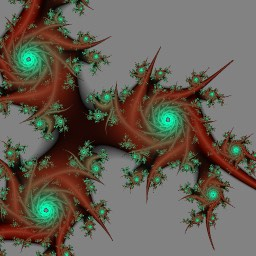algorithms :
• Linear multiply :
In this mode w (the alpha) is multiplied with the RGB directly.
• Power multiply :
In this mode w is raised to the parameter 'power' before the RGB multiplication.
• Gate multiply :
In this mode the alpha is premultiplied by 100 to have a very sharp transition between opaque and transparent regions. When used with "Use depth cue color for alpha" option and the RGB Multi mode you will have transparent regions (alpha<=0) colored with the depth cue color.

Note : the 44 Memory Weighted Add component perform a similar operation for accumulated iterative RGBA data.

##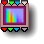44 Alpha Fade

parameters :
A : Feather (0. : 1.)
B : Algorithm dependent

discussion :
44 Alpha Fade uses the alpha channel (w) to blend the first auxiliary color (
Depth cue color) with the RGB channels. 'Feather' parameter controls the rate of the blending : use low value for a sharper transition. When alpha/w is a distance the Exponential algorithm will yield nicer results.
You may use this component to implement a customized depth cuing or to fade a texture into a constant color. The incoming alpha/w is returned unchanged. When alpha is unneeded you have the 43 w Color Fade which provides the same service.

Options (ArtMatic engine 8.06): 'Pass infinity' and 'Discard infinity'. Discarding infinities might be necessary to avoid discontinuities in iterative fractal shaders or to discard the implicit infinity masking. When 'Discard infinity' is set incoming infinities in RGB are shaded with the Depth cue color.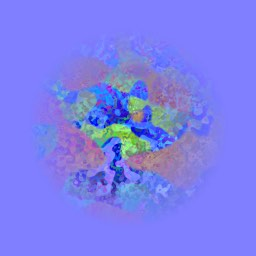algorithms :
• Linear (legacy) :
'Amount' is a multiplier applied to the blending alpha channel. When 'Amount' is positive, the auxiliary color appears where the alpha channel's value is >=0 at a rate depending on 'Feather'. When the value is negative, the auxiliary color appears where the alpha channel is negative.

• Exponential+ :
In this mode fading to depth cue is governed by an exponential which provides more realistic results for haze when w input is the z distance, as provided by ArtMatic 3D objects components. 'Level adjusts' offset moves the point in depth (z) where depth cueing starts.
• Exponential- :
Same as previous but reverses the orientation of fade. Use it when the "far" z value are increasing on negative axis.

##44 Alpha Shade

parameters :
A : Amount (0. : 1.)
B : Alpha contrast (0. : 4.)

discussion :
Alpha Shade shades the w input alpha channel with a gray scale ramp and mixes it with the incoming RGB according to the 'Amount' parameter.

The Alpha Contrast parameter is applied to w before the shading is applied. The alpha channel is also passed out unchanged through the fourth output.

##44 Alpha gradient

parameters :
A : Amount % (0. : 1.)
B : Scale X (0. : 2.)
C : Offset (-2. : 2.)

discussion :
Alpha gradient shades the w input alpha channel with the main Gradient and blend it with the RGB channels. It is similar with the
43 RGB mix but with an alpha output. The alpha channel is also passed out unchanged through the fourth output. 'Amount' controls the mix of the RGB and Alpha/Gradient channel. 'Scale X' and 'Offset' controls the mapping of w to the Gradient : Gradient( Scale* w + Offset). See also 13 Main Gradient if you need more control over how the mapping is performed.

##44 Color Shift

parameters :
A : Hue shift (-6. : 6.)
B : Lightness % (-2. : 2.)
C : Saturation % (0. : 2.)
D : W hue shift % (0. : 1.)

discussion :
Adjust the HLS (Hue, Luminance/Lightness and Saturation) of the RGB image passed into the component. This component is very convenient for adjusting the HLS of an RGB image without having to convert color spaces and can be used with straight RGB images (no alpha) by leaving the fourth input unconnected. Sophisticated color processing effects can be created by adjusting HLS. The output is clipped to a valid color range (i.e. no negative values are passed out). The
Depth cue color is used where the output value is infinity.
The Alpha channel is left unchanged except infinities are discarded and mapped to a alpha transparent value. If you need infinities to be passed on use the 43 w Color Shift or the 33 Color Shift versions.

##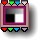44 Contrast & offset

parameters :
A : Color contrast % (0. : 2.)
B : Scale w/alpha (-4. : 8.)
C : Offset w/alpha (-4. : 4.)

##44 Color Controls #

parameters :
Algorithm dependent

discussion :
44 Color Controls # provides the same services as the
33 Color Controls # and passes the alpha channel unchanged.

algorithms :
• Colorize
• Color Floor
• Offset & clip
• RGB Contrast
• Multiply
• MonoChrome
• Invert
• Power Saturation
• Clip and blend

##44 (xziy) City Textures #

parameters :
Algorithm slider : (0 : 7)
B : Color Cycle (0. : 1.)
C : Frequency (0. : 8.)

discussion :
This component is an RGB + Alpha component for creating ArtMatic Voyager terrain-based cityscapes. It provides the color texture for building exteriors and an alpha output that can be used for special effects (like embossing) in ArtMatic Voyager. This component generally provides the color shading for buildings created with the
24 City Maps # (xziy) component and has a special interpretation of the input values.

The inputs are (from left to right):

• x and z. the ground co-ordinates (latitude and longitude)
• i: texture index. Index value that selects the color pattern to use. The color texture for the buildings is determined by the "i" input and not by the ground position. The "i" input will usually be provided by 24 City Maps, but you may pass in a constant (to select a particular texture) or manipulate "i" to select different textures for the same buildings.
• y: elevation.
Output values: The left three outputs are RGB values used as the color texture. The rightmost output (the alpha value) is meant to be used as an extra output in ArtMatic Voyager. It is a 'bump' value that can be used as an 'extra output' value in ArtMatic Voyager (see the image below) rather than an elevation map. The tree below shows a typical use of this component.

The available patterns are:

algorithms :
• City Texture Set A #
• City Texture Set B #
• City Texture Set C #
• City Classic Set #

##44 VY Themes Filters #

parameters :
Algorithm slider : (0 : 9)
B : Level (0. : 1.)
C : Frequency (0. : 8.)

discussion :
This is an RGB+Alpha component used to enhance the visual complexity and realism of terrains for ArtMatic Voyager landscapes and includes vegetation such as grass bushes and trees. The input will be RGB+Alpha and the output is RGB+Alpha.

"Algorithm slider" selects the texture/theme implemented by the component. The "Level" parameter generally shifts the texture vertically but can also modulate the amount or contrast of particular features.

Examples can be found in ..Voyager folder/Voyager Scenes/Theme Filters Scenes.An ArtMatic Terrain passed trough both Erosion & White Rocks & Beach themes filters.

algorithms :
• Gray Rocks # :
• White Rocks & Beach # :
Fractured gray/white rocks and beach with dunes below zero.
• Red Rocks & Beach # :
Fractured rocks and beach below zero. Rocks gets gray.
• Strata Rocks A # :
• Strata Rocks B # :
• Strata Rocks C # :
Stratified patterns with various colors.
• Grass & Bushes # :
Those two filters adds vegetation above 0 and below a certain altitude. The vegetation is of course not realistic but works fairly decently from some distance.
• Pine Forest # :
• Wide Beach, Dunes & Pebbles # :
Adds a beach with dunes & small rocks near 0 but leaves what's high unchanged.
• Erosion # :
Erode the terrain above zero to the level specified by parameter B, (approx. from 500 meter to 2500 meters). It does change the RGB by lowering the brightness inside valleys.

##44 Line shader

parameters :
A : Contrast % (0. : 100.)
B : Width (0. : 5.)
C : Background Color Ramp (-1. : 1.)
D : Contours Color Ramp (-1. : 1.)

discussion :
Takes an RGBA input and assuming Alpha is a balanced DF field Line Shader creates and shades contour line at the Alpha values zero crossings while using a background flat color for negative A values. So practically it is a "contour" shader and will transform any DF functions or any balanced surfaces into a 2D graphic drawings.

The 44 Line shader is similar to 11 line and 21 line and can accommodate high resolution outputs. (See 21 Line discussion). Negative A input values are shaded with the background color taken procedurally from the "Background color ramp" parameter. The color of the Lines (default black) are taken procedurally from the "Contours Color Ramp" parameter. When negative it returns a ramp from white to black (0). When positive it cycles the hues from dark to bright.

44 Line Shader provides an easy way to get a 2D graphic view of a 3D object slice. Here for example is a rendering of almost the same system in 3D and in 2D the only difference being the 2D version uses the 44 line shader at the output of the tree:

Examples:ManyOverlay DF 3D view in Voyager (Voyager Examples/DF modeling/Geometry/Grafic ManyOverlay DF.vy)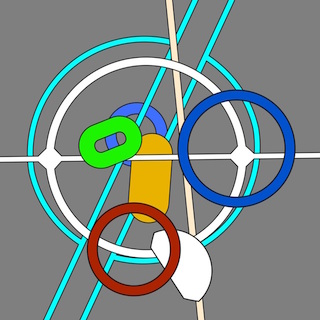##44 Memory Add

parameters :
A : Sum Gain (0. : 1.)
B : Auto Gain % (0. : 1.)

discussion :
Each iteration inputs is accumulated by adding the (x,y,z) and w to the 4D vector output sum. The alpha (w) is added only when positive. In most of the case you will use it to accumulate RGBA data to compose image layers produced iteratively but it can also be used to accumulate any 4D data as the process don't assume inputs represents RGBA data in particular.
When 'Auto Gain' is set at 1 the weight will be calculated using the context iteration max to keep the sum independent of the iteration number. Lower values will blend with the 'Sum Gain' setting. "Memory Add" discards infinities.

##44 Memory Fractal Add

parameters :
A : Power slope (0.06 : 2.)
B : Sum gain (0. : 2.)

##44 Memory Weighted Add

parameters :
A : Alpha Contrast (0. : 2.)
B : Alpha Offset (-2. : 2.)

##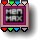44 Memory w Logic #

parameters :
A : Level (-1. : 1.)
B : Smoothness % (0. : 16.)
C : Blend with last % (0. : 1.)
D : Smooth power (0.75 : 2.50)

algorithms :
• Weighted max :
Assign a weight to the input RGBA prior the MAX logic compare. Parameter D (Blend With Last) offers control of the blending between iterations. It is especially valuable when doing fractal iteration. When the parameter is set to the maximum, only the last iteration will be visible leaving only the limit set when working with a fractal.Weighted max - Nfold snowflake uses Weighted max to accumulate objects instances within iterative fractal transforms.

• Maximum :
Parameters : Smoothness , Level
Implement a straight a straight maximum. Max is the logical operation equivalent of Union. 'Level' adjust the level of incoming alpha For each pixel, compare the alpha (w) input value to the stored image's and keep the pixel whose w value is largest. In order to be compatible with DF, the component is initialized to -infinity. You may use it with recursive DF object as an Union logic operator (see 24 Packed Logic)Maximum - Dsks accumulated with the Maximum logic.

• Minimum :
Parameters : Smoothness , Level
Implement a straight minimum. Min is the logical operation equivalent of intersection. 'Level' adjust the level of incoming alpha For each pixel, compare the alpha (w) input value to the stored image's and keep the pixel whose w value is smallest. In order to be compatible with DF, the component is initialized to +infinity.
• Xor :
Parameters : Smoothness Xor sets the intersection of X & Y to Negative. Negative alphas are set to Auxiliary Color A. You may use it with recursive DF object as an Xor logic operator (see 24 Packed Logic)Xor - Disks accumulated with the Xor logic.

• Overlay :
Y shape goes above X shape : successive iteration masked elements will blend above previous elements. Negative alphas are set to Auxiliary Color A.
Parameters : Smoothness, Smooth Power:
As with weighted max 'Smooth Power' scales the smooth value according to iteration level (scaled smooth= smooth/pow(Smooth Power,iteration level). When power is 1 the smooth value is constant. When power is 2 the smooth value gets smaller for higher iterations, while when power is less then 1 smooth gets bigger for higher iterations. Smoothness with Smooth Power can dramatically change the result and can create blur or subtle shadings effects for iterative systems.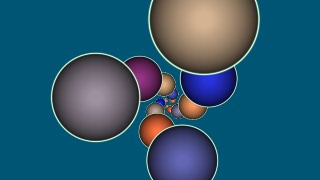Overlay - Disks accumulated with the Overlay logic. Notice the contours are preserved at the intersections unlike with the Maximum logic.

• Underlay :
Y shape goes below X shape : successive iteration masked elements will blend below previous elements. Negative alphas are set to Auxiliary Color A.
Parameters 'Smoothness and 'Smooth Power' works like described above.
"Underlay" is a particularly useful for iterative fractal "Orbit trap" shading as illustrated by the example below:Underlay mode shaded Julia Set (Libraries/Fractales/Mandel OrbitTraps Seed.artm).

##44 Memory w Maths #

parameters :
A : RGB Blend or Smoothness (0. : 1.)
B : RGB Gain % (0. : 1.)
C : Algorithm dependent (0. : 1.)

discussion :
Memory w Maths accumulates RGBA streams using various mathematical functions. Infinity are preserved and incoming infinities in RGB are shaded with the
Depth cue color and alpha is set to transparent.

algorithms :
• Difference :
Subtract each iteration's pixel RGB values from the accumulated image's and returns the Absolute value of the result. Note that since ArtMatic engine 8.06 the resulting Alpha channel is set to the 'smooth' maximum of incoming Alphas.
• RGB Multiply :
Parameters: Smoothness, Gain, Blend
Multiplies RGB on positive inputs and combine the alphas using a smooth max. Blend mixes the result with the incoming RGB. The gain adjust the multiply amplitude.
• Morph :
Parameters : Smoothness
Accumulates the RGBA values using exponentials to blend the alpha values and mixes the colors accordingly. The morph equation is log(exp(x*K)+exp(y*K))/K where K is a smoothing factor. K is derived from the 'Smoothness' parameter. Morph produces a similar result as smooth Max but with a much smoother and natural blending. note: Morph works best with balanced alpha.A fractal using Memory Morph to accumulate various iteration levels.

• Alpha Blend :
Reimplemented in ArtMatic engine 8.06, this algorithm compose successive RGBA inputs using 'Blend Slope' to controls the fading direction. When in middle (0.5) all instances are equals and Alpha Blend behave like standard alpha compositing. When zero higher iterations are faded and reversely at 1 earlier iteration are faded. 'Color Blend' parameter controls how much input color is faded into a constant color using Auxiliary Color A. Leave it at zero for no color fading.
'RGB Gain' adjust the incoming RGB brightness.Alpha Blend with slope above 0.6

• Alpha Blend Max :
Same as above but the resulting alpha will be the maximum of all incoming alpha. This leaves an opaque trace of all iterations.
Example : Libraries/Components demo/MemoryWMAlphaBMAlpha Blend Max with slope above 0.6 and Color Blend at 1.

• DF Union Blend :
This mode is suited for iterative accumulation of DF colored objects, often used in fractals. When parameter C 'Max Alpha Blend' is at 1 the resulting alpha/DF will be the maximum of all incoming alpha/DF. Lower value will shrink the thickness of early DF values, enhancing the latest, usually thinnest, features.
The 'RGB blend' parameter allows to control how much new RGB data is blended into the final sum. Low value will enhance the first RGB values while values close to 1 will give more importance to the latest iterated RGB values.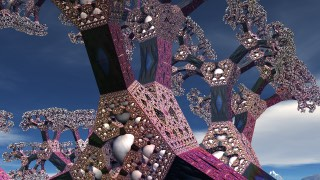DF 3D fractal using 'DF Union Blend'

##44 Memory w Alpha

parameters :
A : Alpha Level (-1. : 1.)
B : Feather (0. : 2.)
C : Start Color (0. : 1.)

discussion :
Memory w Alpha accumulates RGBA streams in iterated systems using alpha channel compositing (alpha value sets the blending amount of incoming RGB with background, zero and below being transparent, 1 being opaque). The 'Start color' sets the starting background color from a procedural color ramp and is visible when the output alpha is ignored in final shader like in RGB Multi
Shading mode. Infinities are discarded : incoming infinities are set to 'Start color' with transparent alpha. The output alpha is computed as the maximum of incoming alphas.
Keep 'feather' at 1 to have a strict alpha blending (alpha values are not scaled).
'Alpha Level' offsets the alpha values for each iterations.
Memory w Alpha has great application for recursive graphics, fractals and multi-layer texturing. For more control on the order of iterative layers compositing you can use the 44 Memory w logic # component with Overlay or Underlay modes as described above.

##44 Packed index (w) Mixer

parameters :
A : Feather % (0. : 1.)
B : Red % (0. : 1.)
C : Green % (0. : 1.)
D : Blue % (0. : 1.)

discussion :
This is a very powerful component that mixes 3 RGBA (or Color/DFRM) inputs using the fourth input to control the mix. This component is generally used with an index value (like the one created by the 24 xyza components or 34 UVID Sweep Volumes) as the fourth input. You may also generate this index with the special logical function
32 Indexed Logic tools # component.
This allows composite objects to be created with different color textures for different parts of the object. Additionally, auxiliary colors can be mapped to some values depending on the parameter option settings.

The output is determined by the index (fourth input) value. Input A is used where the index value is between 0 and 1. Input B is used where the the index is between 1 and 2. Input C is used where the input is between 2 and 3.44 Packed Index Mixer is used to provide different color textures for different tiles.

Depending on various Parameter Options indexes that are outside the range can be mapped in different ways :

• Default
- in this mode index values above 3 are mapped to the parametric color defined by Red Green and Blue. Also, -1 and -2 are mapped to the 2nd and third auxiliary colors.
Inputs over or equal 5 maps to the depth cue color since engine 8.08.
• Clamp to 3
- in this mode, values are clamped to the range from 0 through and including 2.
• Modulo 3 (loop)
- in this mode, the index values are looped from 0 to 2. It can be used with a UVID (see 34 uvid Sweep_Volumes) index to mix several textures and all XYZA tiles (where Z is a texture index).
• modulo 3 with DC (depth cue color)
• modulo 4 with DC (depth cue color)
Both modes maps input >= 5 to the depth cue color and uses a modulo 3 or 4 for the rest of the inputs indexes. With modulo 4 the fourth color is the parametric color of the tile. Inputs over or equal 5 maps to the depth cue color : xyza semiregular patterns and other tiling components often use the value of 5 to separate tiles.

## 44 Compiled Tree :

parameters :
A : Blend (0:1)
B : Recursions

discussion :
Compiled Trees are groups of tiles that can be used in place of single tiles as a kind of macro or subroutine. 44 Compiled Tree with 4 inputs and 4 outputs can process a lot of data at once, in particular if the 4th input is a packed RGBA vector. You can then see the 44 CT as a way to process space + color + alpha data which makes 7 variables, 3 for space and 4 for the RGBA stream. 44 Compiled Trees are often used to create recursive 3D fractal-like objects. For fractal-like objects, the input to the CT will be a 3D space in inputs xyz and the fourth input (w) will be a density field (DF). When using a RGBA stream in the 4Th in/out you can also accumulate an associated RGB fractal texture inside the CT.

usage :
Select a 44 tile and use "New compiled tree" to create a new CT from the selection (Tree Edit menu or type 'n' key).
To save a CT on disk to use the function elsewhere use "Save compiled tree" from the Tree Edit menu.
You may also copy and paste the entire CT by using Copy Tile and Paste Tile from the Edit menu.
44 compiled trees can be made recursive with the option “allow Feedback" ON. That means outputs of the CT will feed inputs after the first iteration. In that case the result will be a recursively transformed 4D space or a transformed 3D space with a packed RGBA stream on output 4. When the option is turned off you will need a memory component at the end inside the CT to accumulate the results. (see
Memory w Logic # discussion).
The fourth input can also be a packed RGBA stream. In that case the first 3 input can just carry the space coordinates that are transformed recursively while the 4th accumulates the RGBA data. This setup allows to create complex colored 3D objects like trees, complex plants or 3D fractals.
Even without recursion, the advantage of using 3D Space + packed RGBA input/output is that you can mix the output with other similar CTs to build up complex plant structures. For example, a first 44 CT might create the first level of branches while a second will add a different secondary branching structure and a third would add the leaves. Each CT will both transform the space for the next and add something in the fourth channel.